##### Question

In: Statistics and Probability

# Our environment is very sensitive to the amount of ozone in the upper atmosphere. The level...

Our environment is very sensitive to the amount of ozone in the upper atmosphere. The level of ozone normally found is 4.5 parts/million (ppm). A researcher believes that the current ozone level is not at a normal level. The mean of 1020 samples is 4.4 ppm. Assume a population standard deviation of 1.2. Does the data support the researcher's claim at the 0.05 level?

Step 1 of 6: State the null and alternative hypotheses.

Step 2 of 6: Find the value of the test statistic. Round your answer to two decimal place

Step 3 of 6: Specify if the test is one-tailed or two-tailed.

Step 4 of 6: Find the P-value of the test statistic. Round your answer to four decimal places.

Step 5 of 6: Identify the level of significance for the hypothesis test.

Step 6 of 6: Make the decision to reject or fail to reject the null hypothesis.

## Solutions

##### Expert Solution

Solution:

Given: The level of ozone normally found is 4.5 parts/million (ppm).

that is: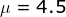Sample Size = n = 1020

Sample mean=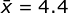Population standard deviation =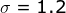Claim:  the current ozone level is not at a normal level. that is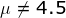Level of significance =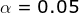Step 1 of 6: State the null and alternative hypotheses.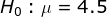Vs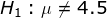Step 2 of 6: Find the value of the test statistic.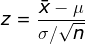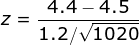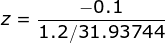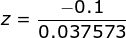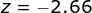Step 3 of 6: Specify if the test is one-tailed or two-tailed.

Since H1 is not equal to type, this is two tailed test.

Step 4 of 6: Find the P-value of the test statistic.

For two tailed test, P-value is given by:

P-value = 2 X P( Z < z ) if z is negative

P-value = 2 X P( Z > z ) if z is positive

P-value = 2 X P( Z < -2.66 )

Look in z table for z = -2.6 and 0.06 and find corresponding area.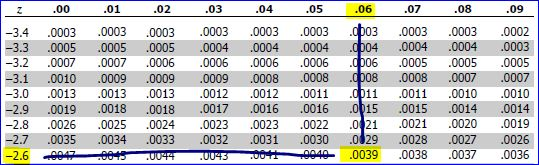P( Z< -2.66) = 0.0039

thus

P-value = 2 X P( Z < -2.66 )

P-value = 2 X0.0039

P-value = 0.0078

Step 5 of 6: Identify the level of significance for the hypothesis test.

Level of significance =Step 6 of 6: Make the decision to reject or fail to reject the null hypothesis.

Decision Rule:
Reject null hypothesis H0, if P-value < 0.05 level of significance, otherwise we fail to reject H0

Since P-value = 0.0078 < 0.05 level of significance, we reject null hypothesis H0.

Thus at 0.05 level of significance, the data support the researcher's claim that  the current ozone level is not at a normal level.

## Related Solutions

##### Our environment is very sensitive to the amount of ozone in the upper atmosphere. The level...
Our environment is very sensitive to the amount of ozone in the upper atmosphere. The level of ozone normally found is 4.14.1 parts/million (ppm). A researcher believes that the current ozone level is at an insufficient level. The mean of 10401040 samples is 4.04.0 ppm. Assume a population standard deviation of 1.21.2. Does the data support the researcher's claim at the 0.020.02 level? Step 1 of 6: State the null and alternative hypotheses. Step 2 of 6: Find the value...
##### Our environment is very sensitive to the amount of ozone in the upper atmosphere. The level...
Our environment is very sensitive to the amount of ozone in the upper atmosphere. The level of ozone normally found is 5.5 parts/million (ppm). A researcher believes that the current ozone level is not at the normal level. The mean of 13 samples is 6.0 ppm with a standard deviation of 0.9 Does the data support the claim at the 0.1level? Assume the population distribution is approximately normal. Step 1 of 3 : State the null and alternative hypotheses. Step...
##### Our environment is very sensitive to the amount of ozone in the upper atmosphere. The level...
Our environment is very sensitive to the amount of ozone in the upper atmosphere. The level of ozone normally found is 5.5 parts/million (ppm). A researcher believes that the current ozone level is not at the normal level. The mean of 13 samples is 6.0 ppm with a standard deviation of 0.9 Does the data support the claim at the 0.1level? Assume the population distribution is approximately normal. Step 1 of 3 : State the null and alternative hypotheses. Step...
##### Our environment is very sensitive to the amount of ozone in the upper atmosphere. The level...
Our environment is very sensitive to the amount of ozone in the upper atmosphere. The level of ozone normally found is 5.5 parts/million (ppm). A researcher believes that the current ozone level is at an excess level. The mean of 6 samples is 5.9 ppm with a variance of 0.49. Does the data support the claim at the 0.05 level? Assume the population distribution is approximately normal. Step 2 of 5 : Find the value of the test statistic. Round...
##### Our environment is very sensitive to the amount of ozone in the upper atmosphere. The level...
Our environment is very sensitive to the amount of ozone in the upper atmosphere. The level of ozone normally found is 4.7 parts/million (ppm). A researcher believes that the current ozone level is at an excess level. The mean of 26 samples is 5.0 ppm with a standard deviation of 0.9. Does the data support the claim at the 0.05 level? Assume the population distribution is approximately normal. Step 5 of 5: Make the decision to reject or fail to...
##### Our environment is very sensitive to the amount of ozone in the upper atmosphere. The level...
Our environment is very sensitive to the amount of ozone in the upper atmosphere. The level of ozone normally found is 4.7 parts/million (ppm). A researcher believes that the current ozone level is at an excess level. The mean of 26 samples is 5.0 ppm with a standard deviation of 0.9. Does the data support the claim at the 0.05 level? Assume the population distribution is approximately normal. Step 4 of 5: Determine the decision rule for rejecting the null...
##### Our environment is very sensitive to the amount of ozone in the upper atmosphere. The level...
Our environment is very sensitive to the amount of ozone in the upper atmosphere. The level of ozone normally found is 7.5 parts/million (ppm). A researcher believes that the current ozone level is at an excess level. The mean of 980 samples is 7.6 ppm. Assume a population standard deviation of 1.1. Does the data support the researcher's claim at the 0.02 level? a) State the null and alternative hypothesis. b) test statistic c) One tailed or two tailed? d)...
##### Our environment is very sensitive to the amount of ozone in the upper atmosphere. The level...
Our environment is very sensitive to the amount of ozone in the upper atmosphere. The level of ozone normally found is 7.0 parts/million (ppm). A researcher believes that the current ozone level is not at a normal level. The mean of 1000 samples is 6.9 ppm. Assume a population standard deviation of 1.2. Does the data support the researcher's claim at the 0.02 level? Step 1 of 5 : State the null and alternative hypotheses. Step 2 of 5 :  Find...
##### Our environment is very sensitive to the amount of ozone in the upper atmosphere. The level...
Our environment is very sensitive to the amount of ozone in the upper atmosphere. The level of ozone normally found is 6.6 parts/million (ppm). A researcher believes that the current ozone level is at an excess level. The mean of 14 samples is 6.7 ppm with a standard deviation of 1.1. Does the data support the claim at the 0.05 level? Assume the population distribution is approximately normal. Step 1 of 5 : State the null and alternative hypotheses.
##### Our environment is very sensitive to the amount of ozone in the upper atmosphere. The level...
Our environment is very sensitive to the amount of ozone in the upper atmosphere. The level of ozone normally found is 7.8 parts/million (ppm). A researcher believes that the current ozone level is at an excess level. The mean of 12 samples is 8.0 ppm with a standard deviation of 0.9. Does the data support the claim at the 0.1 level? Assume the population distribution is approximately normal. Step 4 of 5 :   Determine the decision rule for rejecting the...